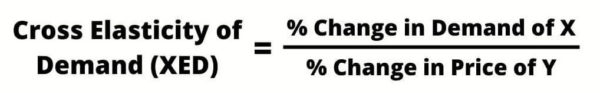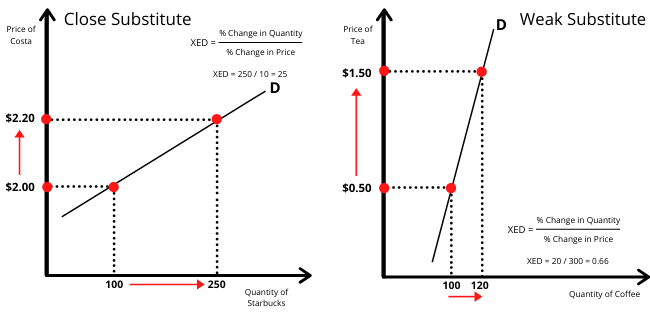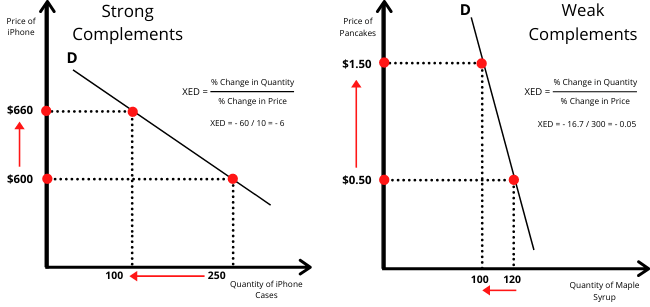# Cross Price Elasticity of Demand

WRITTEN BY PAUL BOYCE | Updated 9 January 2021

## What is Cross Price Elasticity of Demand

Cross Price Elasticity of Demand (XED) measures the relationship between two goods when the price of one changes. So if a Hershey’s chocolate bar increases by 20%, how will that impact on demand for Snickers? In other words, it calculates how the demand for one product is affected by the change in the price of another.

##### Key Points
1. Cross Price Elasticity of Demand measures the relationship between two products and how the price change of one affects the demand of the other.
2. These can be categorised in three types; substitute goods, complementary goods, and unrelated goods.
3. Cross Price Elasticity of Demand can be calculated by dividing change in demand of X by change is price of Y.

Cross Price Elasticity of Demand (XED) covers three types of goods; substitute goods, complementary goods, and unrelated goods. By determining the XED, we can determine the relationship between them.

For instance, two goods with a positive XED are substitute goods. This is because when the price of one good increases, it creates demand for the other good which is now cheaper. By contrast, a complementary good has a negative XED. So when the price of one good goes up, it actually reduces demand for the good being compared.

## Cross Price Elasticity Formula

We can calculate Cross Price Elasticity using the formula:So how does this work in practise? Well let’s take an example:

Let us say that Coca-Cola increase its prices by 10 percent. At the same time, the demand for Pepsi increases by 5 percent. So we divide the change in demand, which in this case is 5 percent; by the change in price, which is 10 percent. Which leaves us with:

XED = 5 percent increase in demand for Pepsi / 10 percent increase in price = 0.5.

That’s very well, but what does that mean? Well the result can come under three categories:

XED > 0 – the two products / services are substitute goods. Which indicates Positive Cross Price Elasticity.

XED < 0 – the two products / service are complementary goods and indicate Negative Cross Price Elasticity

XED = 0 the two products / services are unrelated.

## 3 Types of Cross Price Elasticity

Cross Price Elasticity can come in three forms: positive elasticity, negative elasticity, and unrelated. Let’s look at them below:

### 1. Positive Cross Price Elasticity (Substitutes)

Positive Cross Price Elasticity occurs when the formula produces a result greater than 0. That means that when the price of product X increases, the demand for product Y also increases.

For example, McDonald’s may increase the price of its products by 20 percent. In turn, customers would prefer to go to Burger King as they may offer a cheaper meal. Consequently, Burger King sees a rise in demand of 10 percent. This would suggest that there is a positive relationship between the two.

Positive Cross Price Elasticity is also known as Cross Elasticity of Demand for substitutes. In short, this means that the two goods being compared are substitute products. This can come in the form of close substitutes such as Starbucks and Costa Coffee, or it can come in the form of weak substitutes such as tea and coffee.As we can see from the charts above, there are two types of positive XED. These can come under close substitutes and weak substitutes. Close substitutes are goods that have a strong relationship with each other. So an increase in the price of one good strongly affects the demand of the other.

By contrast, weak substitute goods still have a relationship between them, but it is not substantial. For example, ordinary tea and coffee in the supermarket are not widely exchanged for one another. However, they are substitute goods.

### 2. Negative Cross Price Elasticity (Complementary)

Negative Cross Price Elasticity occurs when the formula produces a result of less than 0. This means that when the price of product X increases, the demand for product Y decreases. In other words, consumers see prices rise of one product and actually buy less of the other product. This is also known as a Complementary Good.

A Complementary Good maybe something like an iPhone and an iPhone case. In essence, a consumer won’t buy an iPhone case without first purchasing an iPhone. So the complementary good is reliant on the other’s demand.

When the price of an iPhone goes up, demand is likely to fall. At the same time, if fewer people are buying iPhones, there will also be fewer people buying iPhone cases.As we can see from the graph above, there are two types of complementary goods – weak complementary and strong complementary. In the first instance, weak complementary goods cover those which rely on each other to be sold, rather than compete against each other. For example, tea and coffee are substitutes as consumers have one or the other. By contrast, a complementary good is one that supplements the other good.

Weak complementary goods are therefore two goods that are used with each other, but not on a frequent basis. For example, pancakes and maple syrup. Other sauces and toppings are used instead, which means the relationship isn’t so strong.

By contrast, strong complementary goods are two products that are largely used together. For instance, iPhones and iPhone cases. As consumers buy fewer iPhones, fewer cases will also be sold.

### 3. Unrelated Cross Price Elasticity

Unrelated Cross Price Elasticity occurs when the formula produces a result of exactly 0. This means that the price of product X can increase by 100 percent, but have no effect of demand for product Y.

When comparing the two products, they have no relationship. For example, we can compare two random products: milk and iPhones. If the price of milk was to increase by 10 percent, it would have no impact on the number of iPhones sold.

## Cross Price Elasticity of Demand Examples

##### Example #1

For this first example, let us compare Pizza Hut and Dominos. After deep consideration, Pizza Hut increases its price by 10 percent. Consumers do not like the price increase and think they are getting ripped off. Consequently, they switch to Dominos, thereby increasing demand by 5 percent.

We need to remember the formula here:

XED = % Change in Demand of X / % Change in Price of Y

So the change in price goes at the bottom and the change in demand goes at the top. Therefore, in this example:

XED = 5 percent increase in demand for Dominos / 10 percent increase in prices for Pizza Hut = 5/10 = 0.5

This would indicate that the relationship between Pizza Hut and Dominos is that of a substitute good. Therefore we can conclude that the relationship is elastic.

##### Example #2

For the second example, let us compare pancakes and maple syrup. The price of pancakes increases by 13 percent. At the same time, demand for maple syrup decreases by 4 percent. This is because consumers buy fewer pancakes, so they also buy fewer complementary products such as maple syrup.

So how does this work into the formula?

XED = -4 percent change in Demand for maple syrup / 13 percent change in price for pancakes = – 4 / 13 = -0.3

Note that the decline in demand for maple syrup is negative, so it must also be included as a negative in the calculation.

As XED is less than 0, it signifies that the relationship has a negative cross price elasticity of demand. In short, pancakes and maple syrup are classified as complementary goods.

##### Example #3

For the third example, we will look at demand at Disneyland Resorts Orlando and Universal Resorts Orlando. Let us say that Disney increases its daily ticket price from \$110 to \$140. As a result, demand at Universal increases from 10,000 to 11,500. Let us now work this out:

Calculating the percent change in Demand:

Difference between New and Old Demand = New Demand: 11,500 – Original Demand: 10,000 = Difference in Demand: 1,500

Percent change in Demand = Difference in Demand: 1,500 / New Demand: 11,500 = 13%

Calculating the percent change in Price:

Difference between New and Old Price = New Price: \$140 – Original Price: \$110= Difference in Price: \$30

Percent change in Price = Difference in Price: \$30 / New Price: \$140 = 21.4%

Calculating XED = 13% / 21.4% = 0.6

So in this example, XED is 0.6, which signals that the two products are substitutes and are relatively elastic.

## Why is Cross Price Elasticity of Demand (XED) useful?

Cross Price Elasticity of Demand is useful because it helps firms shape their strategies. To explain, they can use cross-elasticity to establish a price at which to sell their products. If there are no substitutes, or there is little cross-elasticity with any other product, the firm is able to set prices. Let us look at such factors below:

### 1. Identify complementary products

The firm can potentially use such products to bundle together to create extra demand. For example, most retailers offer a Playstation bundled with games. They usually offer a slight discount over buying them separately, which can help stimulate demand.

### 2. Pricing Strategy

The firm can see how consumers respond to prices. If Company A increases prices and sees the products of Company B increase in demand; it shows that it needs to consider this impact.

### 3. Organizational Strategy

Whether this occurs with complementary products of substitutes; if the firm can identify crucial areas, they may look to integrate. This could come through acquiring or merging with competitors, or through acquiring other firms in the supply chain.

## Cross Price Elasticity of Demand FAQs

How do you calculate cross price elasticity of demand?

Cross Price Elasticity of Demand can be calculated using the formula:

XED = % Change in Demand of X / % Change in Demand of Y

What is cross elasticity of demand with example?

Cross Price Elasticity of Demand (XED) measures the relationship between two goods when the price of one changes. In other words; it calculates how demand for one product is affected by the change in the price of another.

For the second example, let us compare pancakes and maple syrup. The price of pancakes increases by 13 percent. At the same time, demand for maple syrup decreases by 4 percent. This is because consumers buy fewer pancakes, so they also buy fewer complementary products such as maple syrup.
So how does this work into the formula?
XED = -4 percent change in Demand for maple syrup / 13 percent change in price for pancakes = – 4 / 13 = -0.3
Note that the decline in demand for maple syrup is negative, so it must also be included as a negative in the calculation.
As XED is less than 0, it signifies that the relationship has a negative cross-price elasticity of demand. In short, pancakes and maple syrup are classified as complementary goods.

What is cross elasticity of demand and its types?

Cross Price Elasticity of Demand (XED) measures the relationship between two goods when the price of one changes. In other words; it calculates how demand for one product is affected by the change in the price of another.

Cross Price Elasticity can come in three forms: positive elasticity, negative elasticity, and unrelated.Central Bank Definition - A central bank controls the supply of money as well as how it reaches the consumer. It can not only…Price Elasticity of Demand Definition - Price Elasticity of Demand (PED) measures how customers change their behaviour when prices change. In other words, it refers to…Why Free Markets are Important - According to the Collins Dictionary, a free market is an economic system that allows supply and demand to regulate prices,…